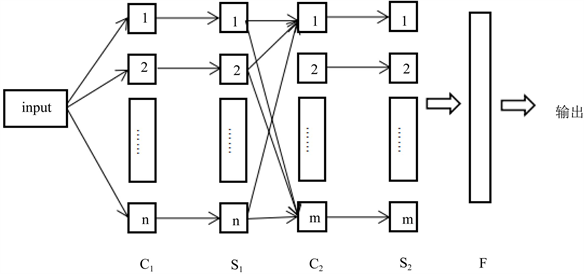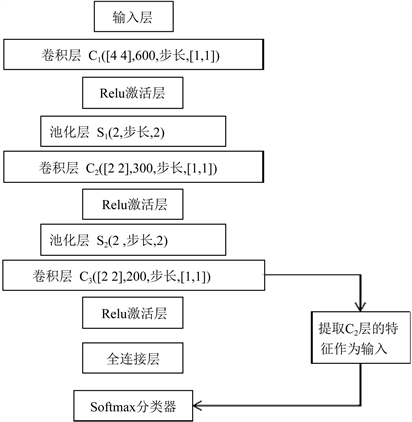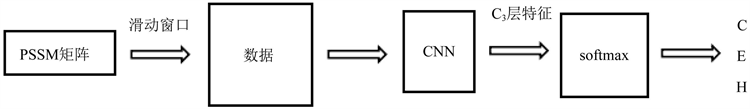# 基于卷积神经网络和Softmax的蛋白质二级结构预测Protein Secondary Structure Prediction Using Convolutional Neural Network and Softmax

• 全文下载: PDF(1013KB)    PP.450-457   DOI: 10.12677/CSA.2019.92051
• 下载量: 314  浏览量: 682   科研立项经费支持

Protein secondary structure prediction belongs to bioinformatics, and it’s important in research area. In this paper, we propose a new prediction way of protein using convolutional neural networks and Softmax. First, the improved convolutional neural network is used to extract the characteristics of the protein amino acid sequence, and then the third convolved output in the convolutional neural network is used as input to the Softmax classifier, and these data are trained and predicted. The dataset is a typical 25PDB dataset for protein. In terms of accuracy, the method is the cross validation based on the 3-fold. The results demonstrate that the accuracy of protein secondary structure prediction is improved.

1. 引言

2. 基于卷积神经网络和Softmax的蛋白质二级结构预测

2.1. 卷积神经网络原理

$s\left(i,j\right)=\left(X*W\right)\left(i,j\right)+b=\underset{k-1}{\overset{n_in}{\sum }}\left({X}_{k}*{W}_{k}\right)\left(i,j\right)+b$ (1)Figure 1. Convolutional neural network structure

2.2. Softmax回归模型

${h}_{\theta }\left(x\right)=\frac{1}{1+\mathrm{exp}\left(-{\theta }^{T}x\right)}$ (2)

$J\left(\theta \right)=-\frac{1}{m}\left[\underset{i=1}{\overset{m}{\sum }}{y}^{\left(i\right)}\mathrm{log}{h}_{\theta }\left({x}^{\left(i\right)}\right)+\left(1-{y}^{\left(i\right)}\right)\mathrm{log}\left(1-{h}_{\theta }\left({x}^{\left(i\right)}\right)\right)\right]$ (3)

${h}_{\theta }\left({x}^{\left(i\right)}\right)=\left[\begin{array}{c}p\left({y}^{\left(i\right)}=1|{x}^{\left(i\right)};\theta \right)\\ p\left({y}^{\left(i\right)}=2|{x}^{\left(i\right)};\theta \right)\\ ⋮\\ p\left({y}^{\left(i\right)}=k|{x}^{\left(i\right)};\theta \right)\end{array}\right]=\frac{1}{{\sum }_{j=1}^{k}{e}^{{\theta }_{j}^{T}{x}^{\left(i\right)}}}\left[\begin{array}{c}{\theta }_{1}^{T}{x}^{\left(i\right)}\\ {\theta }_{2}^{T}{x}^{\left(i\right)}\\ ⋮\\ {\theta }_{k}^{T}{x}^{\left(i\right)}\end{array}\right]$ (4)

$\theta =\left[\begin{array}{c}—{\theta }_{1}^{T}—\\ —{\theta }_{2}^{T}—\\ ⋮\\ —{\theta }_{K}^{T}—\end{array}\right]$ (5)

Softmax回归所对应的损失函数如下所示：

$J\left(\theta \right)=-\frac{1}{m}\left[\underset{i=1}{\overset{m}{\sum }}\underset{j=1}{\overset{k}{\sum }}1\left\{{y}^{\left(i\right)}=j\right\}\mathrm{log}\frac{{e}^{{\theta }_{j}^{T}{x}^{\left(i\right)}}}{{\sum }_{l=1}^{k}{e}^{{\theta }_{l}^{T}}{x}^{\left(i\right)}}\right]$ (6)

$P\left({y}^{\left(i\right)}=j|{x}^{\left(i\right)};\theta \right)=\frac{{e}^{{\theta }_{j}^{T}{x}^{\left(i\right)}}}{{\sum }_{l=1}^{k}{e}^{{\theta }_{j}^{T}{x}^{\left(i\right)}}}$ (7)

2.3. 卷积神经网络和Softmax网络模型

3. 实验和分析Figure 2. Convolutional neural network-Softmax network structure

3.1. 蛋白质数据库

3.2. 实验过程Figure 3. Experimental flow chart

3.3. 实验结果及分析

Q3是被用于残基上的，通过计算正确预测的蛋白质残基占已知蛋白质二级结构序列中总的残基数的比例计算出。Q3值的范围为 $\left[0,1\right]$ ，1表示准确预测。Q3就可以表示为：

${Q}_{3}=\frac{正确预测的残基数}{残基总数}$ (8)

SOV方法是由Burkhard Rost  等人提出的一个基于重叠片段比值的测度。与Q3正确率的计算方法不同的是，SOV方法计算的是能够正确预测蛋白质二级结构的片段比例，从影响因素的角度来看，SOV会忽略一些蛋白质二级结构元素末端的小错误。Table 1. The results of convolutional neural networkTable 2. The results of convolutional neural network-Softmax

4. 总结

  Dulbecco, R. (1986) A Turning Point in Cancer Research: Sequencing the Human Genome. Science, 231, 1055-1057. https://doi.org/10.1126/science.3945817  Zvelebil, M.J. and Baum, J.O. (2007) Understanding Bioinformatics. Garland Sci-ence, USA. https://doi.org/10.1201/9780203852507  岳俊杰, 冯华, 梁龙. 蛋白质结构预测实验指南[M]. 北京: 化学工业出版社, 2010.  Kaufman, L. and Rousseeuw, P.J. (2009) Finding Groups in Data: An Introduction to Cluster Analysis. John Wiley & Sons, New York.  Huang, Z. (1997) Clustering Large Data Sets with Mixed Numeric and Categorical Values. Proceedings of the 1st Pacific-Asia Conference on Knowledge Discovery and Data Mining (PAKDD), 21-34.  LeCun, Y., Bengio, Y. and Hinton, G. (2015) Deep Learning. Nature, 521, 436. https://doi.org/10.1038/nature14539  曲建岭, 杜辰飞, 邸亚洲, 等. 深度自动编码器的研究与展望[J]. 计算机与现代化, 2014(8): 128-134.  张阳, 刘伟铭, 吴义虎. 基于深信度网络分类算法的行人检测方法[J]. 计算机应用研究, 2016, 33(2): 594-597.  LeCun, Y., Bottou, L., Bengio, Y., et al. (1998) Gradient-Based Learning Applied to Document Recognition. Proceedings of the IEEE, 86, 2278-2324. https://doi.org/10.1109/5.726791  Memisevic, R., Zach, C., Pollefeys, M., et al. (2010) Gated Softmax Classification. Advances in Neural Information Processing Systems, 1603-1611.  Long, J., Shelhamer, E. and Darrell, T. (2017) Fully Convolutional Networks for Semantic Segmentation. IEEE Transactions on Pattern Analysis and Machine Intelligence, 39, 3431-3440.  Hubel, D.H. and Wiesel, T.N. (1962) Receptive Fields, Binocular Interaction and Functional Architecture in the Cat's Visual Cortex. The Journal of Physiology, 160, 106-154. https://doi.org/10.1113/jphysiol.1962.sp006837  Hosmer Jr., D.W., Lemeshow, S. and Sturdivant, R.X. (2013) Applied Lo-gistic Regression. John Wiley & Sons, New York. https://doi.org/10.1002/9781118548387  范永东. 模型选择中的交叉验证方法综述[D]: [硕士学位论文]. 太原: 山西大学, 2013.  Altschul, S.F., Gish, W., Miller, W., et al. (1990) Basic Local Alignment Search Tool. Journal of Molecular Biology, 215, 403-410. https://doi.org/10.1016/S0022-2836(05)80360-2  邹权, 郭茂祖, 韩英鹏, 等. 多序列比对算法的研究进展[J]. 生物信息学, 2010, 8(4): 311-315.  Zemla, A., Venclovas, Č., Fidelis, K., et al. (1999) A Modified Definition of Sov, a Segment-Based Measure for Protein Secondary Structure Prediction Assessment. Proteins: Structure, Function, and Bioinformatics, 34, 220-223. https://doi.org/10.1002/(SICI)1097-0134(19990201)34:2<220::AID-PROT7>3.0.CO;2-K